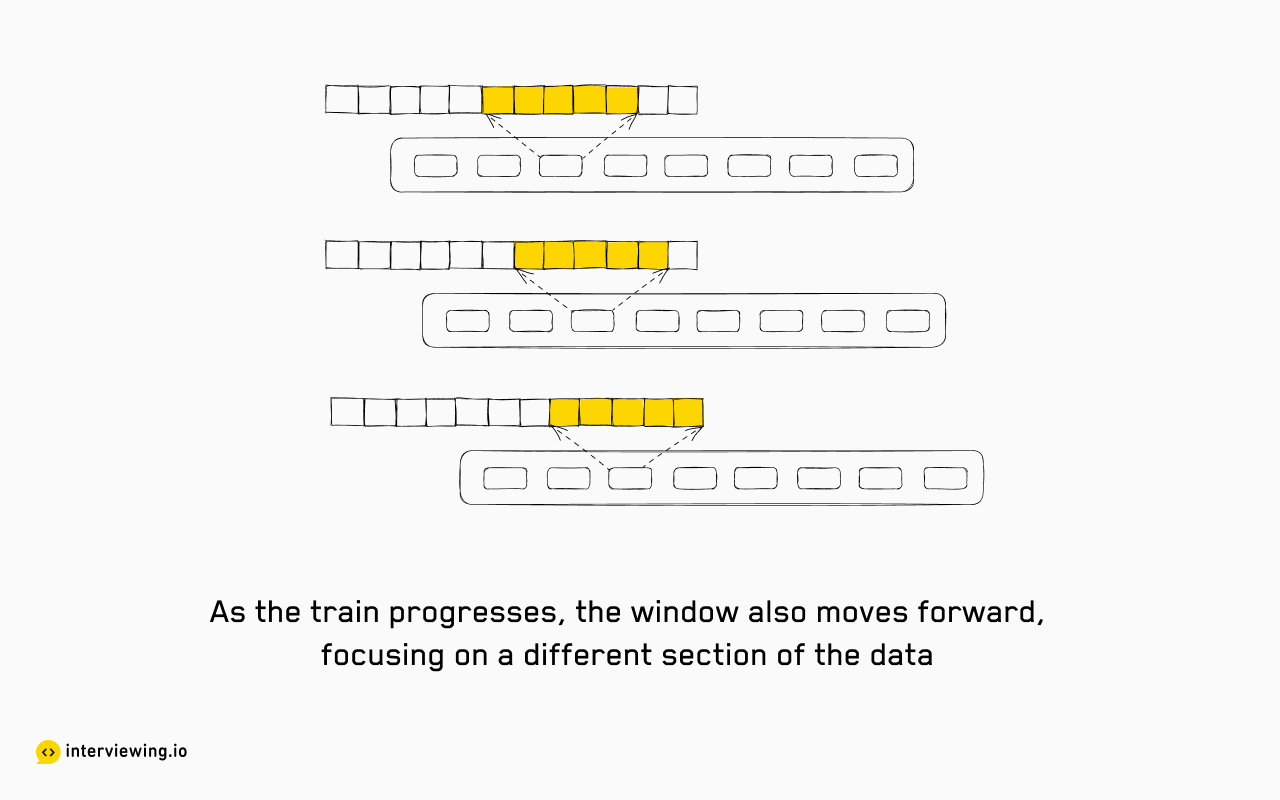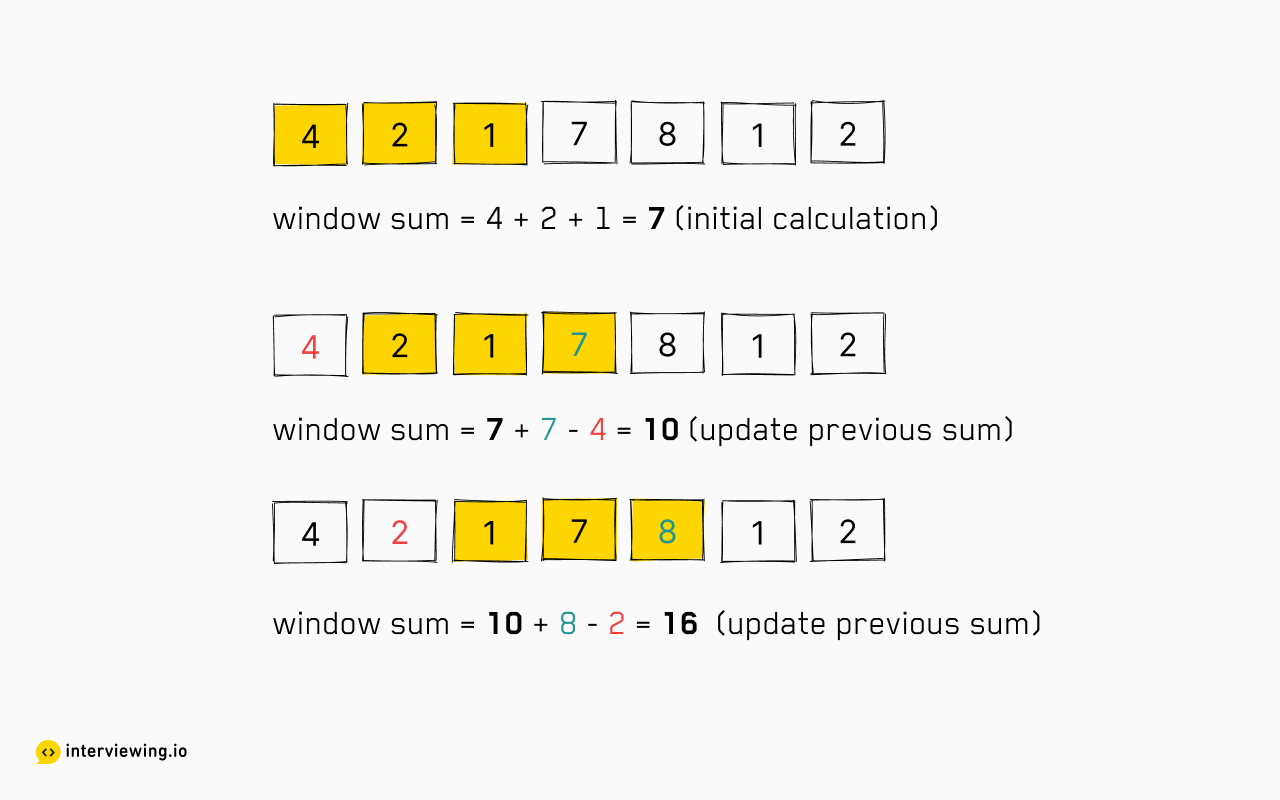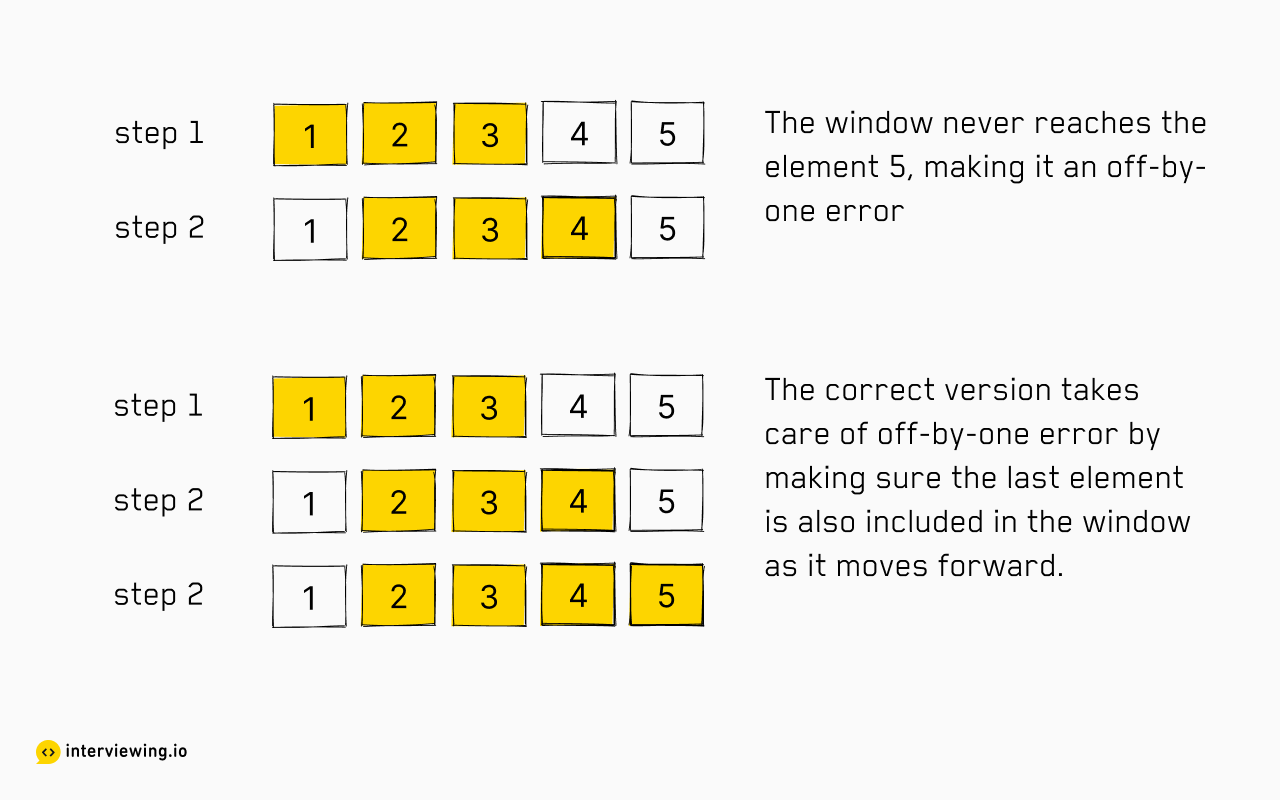# Sliding Window Interview Questions & Tips

By Jai Pandya | Published: July 5, 2023

The sliding window technique is a powerful and frequently used algorithmic technique in coding interviews. It offers an elegant way to solve a broad array of problems, often related to subarrays or substrings, with maximum efficiency. This article aims to provide a deep dive into this technique, highlighting when it's beneficial, common pitfalls, and how to demonstrate proficiency in interviews.

## What is a Sliding Window?

The sliding window technique provides an efficient method for handling subarrays and substrings. It is a specialized subtype in the broader umbrella of Two Pointer problems. This approach involves creating a 'window' that slides over the data to compute a desired result. It uses the result of the previous window position to calculate the result of the position of the window. Sliding Window is potentially one of the most common patterns you'll come across during a coding interview to solve array or string-related questions.

Picture yourself aboard a train, where the view from your window continually changes. You can see a part of the scenery outside, but as the train advances, the portion of the scene you can observe changes. You're not moving within the train; instead, the train (and thus the window) is moving over the landscape.

This is precisely how the sliding window technique works in algorithmic problems. Consider the 'landscape' as your array or list of data. The 'train window' is your 'sliding window', which moves over the data, taking a subset of it into consideration at any given moment. As the window slides over the data, it helps to focus on a specific portion, analyze it, and find particular properties of the elements in that section.The brilliance of the sliding window technique comes from its strategy of decomposing a larger problem into smaller, manageable sub-problems.

For instance, consider the problem of finding subarray sums of size `k` for an array. A straightforward interpretation might view it as `n` separate problems of adding up `k` elements. However, the sliding window technique reframes this into a single, flowing problem. As the window of size `k` slides over the array, the sum of the `k` elements is continually adjusted by adding the new incoming element and subtracting the outgoing one. In this way, a larger problem—is decomposed into a simpler, ongoing task —updating a single sum as the window slides. This approach significantly reduces redundant computations and enhances efficiency.The technique is especially beneficial when the solution can be iteratively constructed from contiguous sections of larger data sets, where the order of data plays a crucial role, such as strings, arrays, or linked lists. The advantage of the sliding window is its efficiency—often bringing the complexity down to `O(n)`.

## When to Use Sliding Window

The Sliding Window technique is a great fit for problems where you're working with linear data structures like arrays or strings, and you need to find something specific within a subarray or substring. This "something specific" could be a maximum or minimum value, a target sum, or a specific pattern. Essentially, if your problem involves sequentially scanning through the data and the task is focused on contiguous portions of this dtaa, the Sliding Window technique can often provide an efficient solution.

It's important to note here the distinction between subarrays and subsequences. Subarrays and substrings refer to contiguous segments of the original data. For example, in the array `[1, 2, 3, 4, 5]`, `[1, 2, 3]` and `[4, 5]` are subarrays—notice how the elements are adjacent to each other. On the other hand, subsequences can contain elements that are not contiguous. In the same array `[1, 3, 5]` is a subsequence—the elements are not adjacent but they maintain the original order. To further clarify, the Sliding Window technique lends itself well to the "Longest Common Substring" problem, but it is not suitable for the "Longest Common Subsequence" problem due to the non-contiguous nature of subsequences.

## How to Use Sliding Window in an Interview

There are two main types of sliding window problems: fixed-size and variable-size sliding windows. The distinction lies in whether the window's size remains constant as it slides or changes based on certain conditions. In this section, we'll see how to work with both types.

### Fixed-Size Sliding Window

In a fixed-size sliding window problem, we maintain a window of a fixed-size 'k' that slides through the data structure.

#### Approach

1. First, we compute the desired result (like sum, average, count) for the initial size 'k' window.
2. Then, we slide the window one element at a time. For every slide, we adjust our result by adding a new element and removing the last element of the previous window.
3. While sliding the window, we keep track of the desired outcome (like maximum sum, longest sequence, and minimum average).

This approach can be seen in many fixed-size sliding window problems.

#### Example

Given an integer array `nums`, find a contiguous subarray whose length is 'k' with the maximum/minimum average value. Also, output the maximum/minimum average value.

#### Solution

We use a fixed-size sliding window to tackle this problem. After initializing variables for window start and window sum, we calculate the sum of the initial window size 'k'. Moving the window by one element at each step, we adjust our window sum by subtracting the outgoing element and adding the incoming element. We continuously calculate the average and track the maximum average found so far.

``````def find_max_average(nums, k):
window_start, window_sum = 0, 0.0
max_avg = float('-inf')

for window_end in range(len(nums)):
# Add the incoming element to the window sum
window_sum += nums[window_end]
# If we've hit the window size, start sliding
if window_end >= k - 1:
# Calculate average of current window and compare with max_avg
max_avg = max(max_avg, window_sum / k)
# Subtract the outgoing element from window sum
window_sum -= nums[window_start]
# Slide the window
window_start += 1

return max_avg``````

### Variable-Size Sliding Window

In variable-size sliding window problems, the window's size changes based on certain conditions.

#### Approach

2. Expand the window until it no longer satisfies the problem's condition.
3. Contract the window from the left, continuously checking if it satisfies the condition.
4. Repeat expanding and contracting the window while keeping track of the minimum/maximum size or other desired outcomes.

This approach can be generalized for a variety of variable-size sliding window problems.

#### Example

Longest Substring Without Repeating Characters: Given a string, find the length of the longest substring without repeating characters.

#### Solution

This is a variable-size sliding window problem. We maintain a dictionary to keep track of the characters and their latest indices in the window. We expand the window from the right, adding the rightmost character to the window. If this character is already in the dictionary (which means it's a repeated character), we slide the window start to the right of the previous occurrence of the character. This way, we ensure the window always contains unique characters. Meanwhile, we continuously calculate and track the maximum length of substrings we've found so far.

``````def length_of_longest_substring(s):
window_start, max_length = 0, 0
char_index_map = {}

for window_end in range(len(s)):
right_char = s[window_end]

if right_char in char_index_map:  # If the character is repeated in the window
# Slide the start of the window to the right of
# the previous occurrence of the right_char
window_start = max(window_start, char_index_map[right_char] + 1)

char_index_map[right_char] = window_end  # Store the current index of the character

# Compute the current window size and compare with max_length
max_length = max(max_length, window_end - window_start + 1)

return max_length
``````

## Common Mistakes in Interviews Featuring Sliding Window

Understanding common pitfalls can significantly improve your performance in interviews. Here are some to watch out for when employing the Sliding Window technique:

### Off-by-One Errors

Off-by-one errors, a common stumbling block in programming, occur when an element is missed or processed more than necessary due to an incorrect condition in the loop or a wrongly set boundary. In the context of the sliding window technique, this often involves miscalculating the window's start or end indices.

Let's take an example. Suppose we have an array `nums = [1, 2, 3, 4, 5]` and we need to compute the sum of every subarray of size 3. A common off-by-one mistake would be incorrectly setting the loop condition, leading to missing an entire window:

``````window_size = 3
for i in range(len(nums) - window_size):  # Off-by-one error
print(sum(nums[i: i + window_size]))
``````

In this example, the loop terminates one step earlier than required, so the sum for the last window `[3, 4, 5]` is never computed. The correct approach would be to adjust the loop to `range(len(nums) - window_size + 1)`.To avoid such off-by-one errors, always carefully consider the indices of your window and ensure that your loop conditions accommodate the entire array or list. It's beneficial to dry-run your code with simple test cases to ensure you aren't missing any elements or windows.

### Jumping Directly to the Optimized Solution

A common mistake during interviews is jumping directly to the most optimized solution. While it's important to aim for optimization, it's equally crucial to demonstrate your problem-solving journey to the interviewer. Start with a simple brute force solution, highlighting its inefficiencies, and discuss why a more optimal solution is required. Then, introduce the Sliding Window technique as a more efficient alternative, illustrating the improvements in time complexity.

For instance, in a problem where you have to find a maximum sum subarray of a specific size `k`, you might say, "An initial brute force approach could involve generating all possible subarrays of size `k` and calculating their sums. This would lead to a time complexity of `O(n*k)`. By implementing the Sliding Window approach, we can maintain a running sum within a 'window' of elements, sliding this window across our array to find subarray sums in `O(n)` time, significantly improving our algorithmic performance."

### Overlooking Edge Cases and Not Asking Clarifying Questions

While dealing with sliding window problems, it's essential to consider edge cases that could lead to bugs or incorrect answers. Edge cases are unusual or extreme situations, such as an empty input, a single-element array, or a window size larger than the array itself. Handling these scenarios appropriately in your code is crucial.

Asking insightful, clarifying questions during the process of understanding the problem can help you identify edge cases. Let's consider the problem "Find the longest substring with at most `k` distinct characters".

To start, you might ask, "Are upper and lower case characters considered distinct or the same for this problem?"

Then, you could inquire about the nature of `k` as well. For example, you might ask, "Can `k` be zero? If so, should the function return an empty string?", or "Can `k` be negative?". You could also ask, "What should happen if the string is empty?"

By asking these questions, you demonstrate a comprehensive understanding of the problem, a keen eye for edge cases, and a desire to produce an accurate, optimized solution. This approach ensures you understand all facets of the problem before diving into code, potentially saving you from having to backtrack.

### Not Testing Enough

The insights from understanding edge cases and asking clarifying questions should inform the tests you use to validate your code. After coding your solution, you should conduct dry runs with a variety of test cases. This includes 'normal' scenarios, edge cases that you've identified, and scenarios based on the clarifying questions you've asked.

For instance, if you've handled a case in your code where `k` can be zero or negative, ensure to include these situations in your tests. Similarly, if you've considered scenarios where the input string is empty, your tests should reflect this as well.

This phase of testing your solution validates your code and demonstrates to your interviewer that you understand the importance of thorough testing and quality assurance.

### Writing Messy Code

Clear, well-structured code signals your expertise. When applying the sliding window technique, your code should accurately represent the “window”. Use descriptive variable names like 'window_start' and 'window_end' instead of 'i' and 'j'. Be mindful of potential off-by-one errors when moving the window or updating its state.

``````window_start, max_length = 0, 0
for window_end in range(len(input)):
# Code to update the window's state goes here...
while # condition to shrink the window:
# Code to shrink the window goes here...
window_start += 1``````

### Neglecting Complexity

It's crucial to discuss the time and space complexity of your solution. Ideally, the sliding window portion of your solution should aim for `O(n)` time complexity since each element in the array or string should be visited only once by the 'window'. However, remember that other factors and operations, such as sorting an unsorted array before applying the sliding window, may increase the overall time complexity.

As you discuss complexity, don't forget to consider any auxiliary data structures, like dictionaries, used in your solution. These contribute to space complexity, which is also an important part of the efficiency of your code. For instance:

``````# For example, this dictionary contributes to space complexity:
char_index_map = {}``````

### Overlooking Improvements

Lastly, discuss how your solution could bbe further optimized or adapted to different problems. For instance, if the input only contains certain characters, you could use a fixed-size array instead of a dictionary to track the window's contents.

``````# If the input only contains ASCII characters:
window_contents =  * 128
``````

## Adjacent Topics to Sliding WindowJai Pandya

Jai is a software engineer and a technical leader. In his professional career spanning over a decade, he has worked at several startups and companies such as SlideShare and LinkedIn. He is also a founder of a saas product used by over 10K companies across the globe. He loves teaching and mentoring software engineers. His mentees have landed jobs at companies such as Google, Facebook, and LinkedIn.